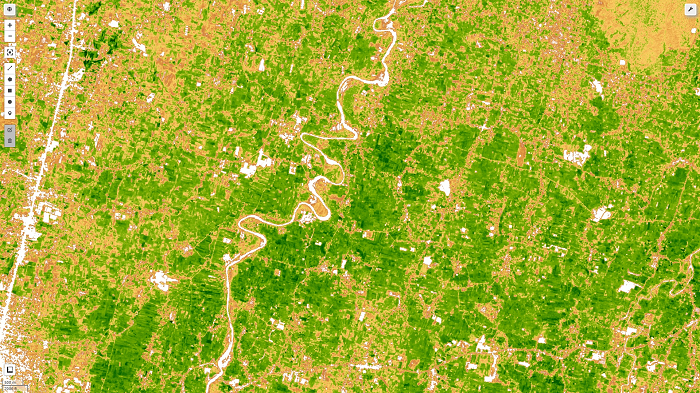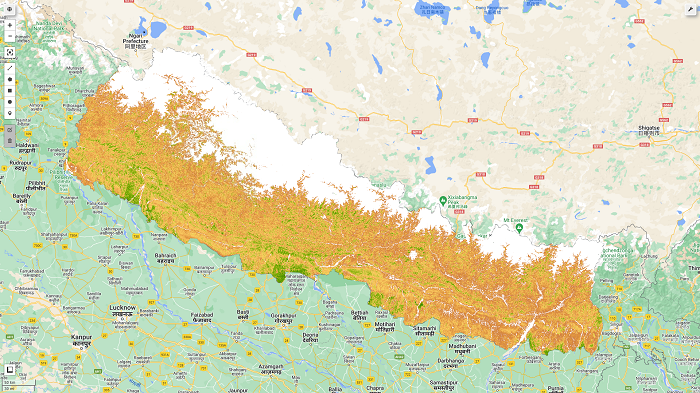# Retrieving Leaf Area Index (LAI) on Sentinel 2 image with Google Earth Engine (GEE)# What is Leaf area index (LAI)?

The leaf area index (LAI), equal to half of the total green leaf area per unit of the horizontal ground surface, is a crucial structural feature of vegetation. Canopy interception, evapotranspiration, and gross photosynthesis are directly related to LAI since leaf surfaces constitute the primary frontier of energy and mass exchange.

I have explained this on my previous post, but I thought it needs to be covered in sperate post along with step by step guide for computation of LAI. I have uploaded the notebook for it's implementation at the end of this article.

## Leaf Area Index (LAI) Formula:

This index is used to forecast crop growth and yield and estimate foliage cover. ENVI calculates green LAI using the empirical formula developed by Boegh et al. (2002):

``````LAI = (3.618*EVI - 0.118)

EVI is Enhanced Vegetation Index value``````

Details for calculation of EVI is here in my previous post

LAI values  ranges typically from 0 to 3.5.

``Leaf area index (LAI) value range: 0 to 3.5``

The LAI values can approach 3.5 when the scene comprises clouds and other bright objects that cause saturated pixels. Before making an LAI image, you should mask clouds and bright features from your scene.

# Calculation of LAI for Sentinel 2 in Google Earth Engine (GEE):

Now, we go one by one step for computation of LAI on Google Earth Engine with python API:

1. Import earth engine api, geemap and authenticate GEE

``````# Import earth engine python api and geemap
import geemap, ee

# Authenticate the earthengine with credentials
ee.Initialize()``````
2. Provide area of interest for calculation
``````# get our Nepal boundary
# I have taken level 0 data for country data from FAO datasets
aoi = ee.FeatureCollection("FAO/GAUL/2015/level0") \
3. Define function for EVI calculations
``````def getEVI(image):
# Compute the EVI using an expression.
EVI = image.expression(
'2.5 * ((NIR - RED) / (NIR + 6 * RED - 7.5 * BLUE + 1))', {
'NIR': image.select('B8').divide(10000),
'RED': image.select('B4').divide(10000),
'BLUE': image.select('B2').divide(10000)
}).rename("EVI")

return(image)``````
4. Date of image, addtion in image collection as a properties
``````def addDate(image):
img_date = ee.Date(image.date())
img_date = ee.Number.parse(img_date.format('YYYYMMdd'))
5. Filter image and apply EVI computation formula
``````# filter sentinel 2 images and apply the EVI formula, finally we obtain
# single image with median operation

Sentinel_data = ee.ImageCollection('COPERNICUS/S2') \
.filterDate("2022-03-01","2022-03-31").filterBounds(aoi) \
.map(getEVI)``````
6. Computation of EVI
``````def getLAI(image):
LAI = image.expression(
'(3.618*EVI - 0.118)', {
'EVI': image.select('EVI')
}).rename("LAI")

return(image)``````

We will be using formula: LAI = (3.618*EVI - 0.118) for LAI

7. Apply LAI computation function to the image

``Lai_image = Sentinel_data.map(getLAI).map(addDate).median().clip(aoi)``
8. Add layers to map and visualize it.
``````# set some thresholds
#  A nice EVI palette
palett = [
'FFFFFF', 'CE7E45', 'DF923D', 'F1B555', 'FCD163', '99B718',
'74A901', '66A000', '529400', '3E8601', '207401', '056201',
'004C00', '023B01', '012E01', '011D01', '011301']

pall = {"min":0.5, "max":3.5, 'palette':palett}
map1 = geemap.Map()
map1.centerObject(aoi, 8)

map1``````

The expected output for this tutorial as shown in the figure below:Please let us know your thoughts on this with following comment section we will definetly reach out to you on your query and feedback.

##### Jorge Conrado Conforte | View the Lai figure

Feb. 9, 2023, 1:28 p.m.

Hi, I'm new geemap, I run your script LAI_calculations.py. Please, how can I visualize and save the Lai figure. Thanks, Conrado

##### Krishna Kafle | Visualization of LAI figure

Feb. 11, 2023, 5:48 a.m.

Hello, thanks for the question. I have already shared the notebook for visualization for this tutorial. You will be able to visualize the LAI figures on jupyter notebook. Please run this, you will get your requirements: https://github.com/krishnakafle/Blog_kaflekrishna/tree/main/GEE/LAI

##### Felipe Alves Ferreira | Calculation of LAI using Landsat 7 with Google Earth Engine

Feb. 26, 2023, 3:47 a.m.

Hi, I'm Just about to ask If It's possible to using The same logic that u guys are using for sentinel, if I can apply to landsat image séries! I want to try to use this method with data time series!